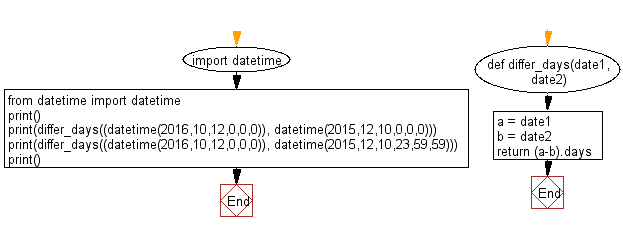﻿ Python: Calculate number of days between two datetimes - w3resource# Python: Calculate number of days between two datetimes

## Python Datetime: Exercise-33 with Solution

Write a Python program to calculate number of days between two datetimes.

Sample Solution:

Python Code:

``````import datetime
from datetime import datetime

def differ_days(date1, date2):
a = date1
b = date2
return (a-b).days
print()
print(differ_days((datetime(2016,10,12,0,0,0)), datetime(2015,12,10,0,0,0)))
print(differ_days((datetime(2016,10,12,0,0,0)), datetime(2015,12,10,23,59,59)))
print()
```
```

Sample Output:

```307
306
```

Flowchart:## Visualize Python code execution:

The following tool visualize what the computer is doing step-by-step as it executes the said program:

Python Code Editor:

What is the difficulty level of this exercise?

Test your Python skills with w3resource's quiz

﻿

## Python: Tips of the Day

Free up Memory:

• Manual garbage collection can be performed on timely or event based mechanism.
```import gc
collected_objects = gc.collect()
```